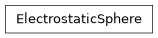# geoana.em.static.ElectrostaticSphere#class geoana.em.static.ElectrostaticSphere(radius, sigma_sphere, sigma_background, primary_field=None, location=None)#

Class for electrostatic solutions for a sphere in a wholespace.

The `ElectrostaticSphere` class is used to analytically compute the electric potentials, fields, currents and charge densities for a sphere in a wholespace.

Parameters:

sigma_spherefloat

conductivity of target sphere (S/m).

sigma_backgroundfloat

background conductivity (S/m).

primary_field(3) array_like, optional

amplitude of primary electric field. Default is (1, 0, 0).

location(3) array_like, optional

center of the sphere. Default is (0, 0, 0).

Attributes

 `location` Center of the sphere. `primary_field` Amplitude of the primary current density. `radius` Radius of the sphere in meters. `sigma_background` Electrical conductivity of the background in S/m. `sigma_sphere` Electrical conductivity of the sphere in S/m.

Methods

 `charge_density`(xyz[, dr]) charge density on the surface of a sphere in a uniform wholespace `current_density`(xyz[, field]) Current density for a sphere in a uniform wholespace `electric_field`(xyz[, field]) Electric field for a sphere in a uniform wholespace `potential`(xyz[, field]) Electric potential for a conductive sphere in a uniform electrostatic field.